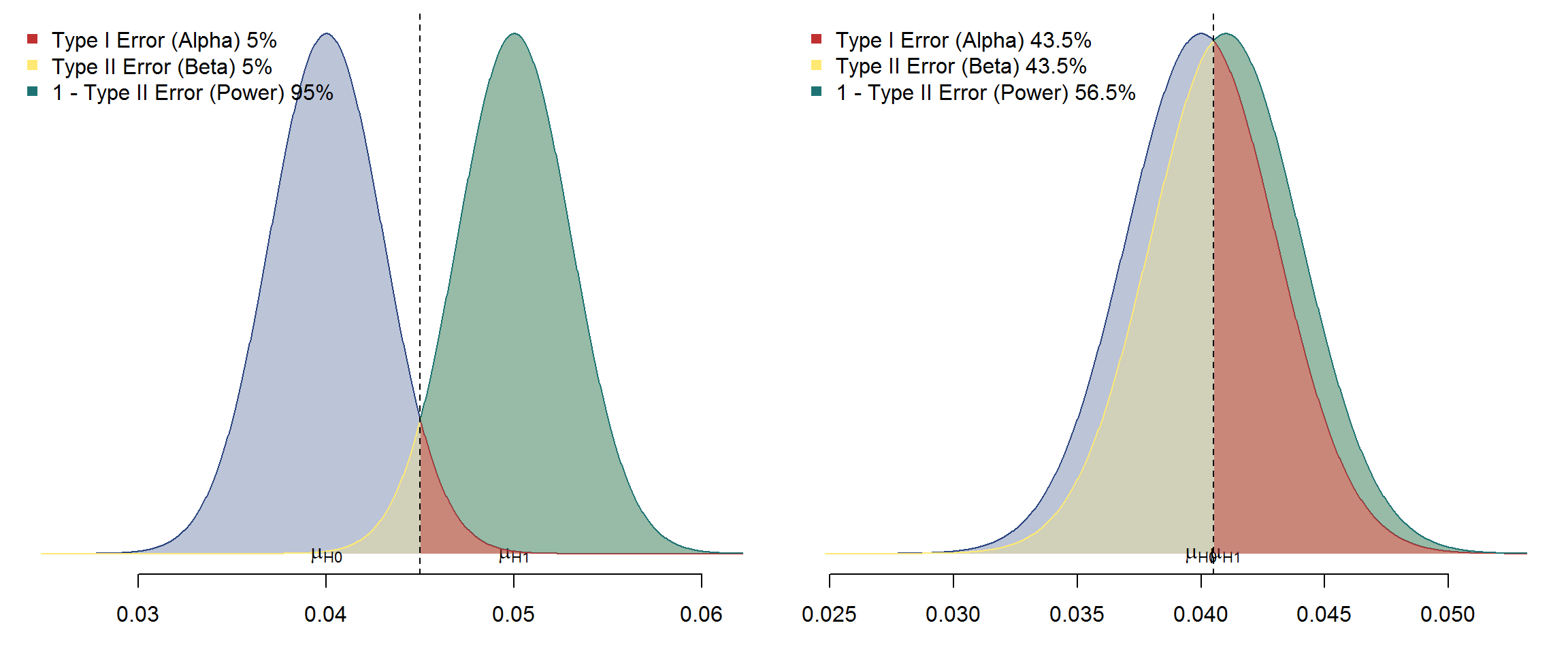# t tests and classifiers

On March 10th, 2016 Keyvan asked the following question on researchgate:

### “Is it true to say that if Two-sample t-test shows that two data are significantly different, then they are good feature for classification purposes?”

Alas, the answer is not necessarily (in fact, more likely not) ! Well,it all depends on what you expect from a “good” feature.

The problem is the aggregate nature of the t.test: it simply detects differences in the estimated means of the two populations. For large enough sample sizes, even with very heavy overlap in the two distributions the t test will be significant but the separation of the individual data points is poor!

Even though we are not in the hypothesis test setting, it is helpful to define the discriminative power of a feature by its respective type-I and type-II errors.

Remember, with any decision rule we can be wrong in two different ways:

• Type I error: We reject the Null hypothesis, even though it is true. This error rate is denoted by $$\alpha$$.
• Type II error: We fail to reject the Null hypothesis, even though it is wrong. This error rate is denoted by $$\beta$$.

A closely related concept is the power of a test. All three measures are visualized in the following two plots where we choose a symmetric classification rule ($$N=10^3$$):I would expect us to conclude that the left panel shows a (very) good feature for classification purposes whereas the right panel not at all.

Would you be surprised to learn that in both cases the t tests are highly significant? Here it is for the left panel

##
##  Welch Two Sample t-test
##
## data:  s1 and s2
## t = -74.234, df = 1995.2, p-value < 2.2e-16
## alternative hypothesis: true difference in means is not equal to 0
## 95 percent confidence interval:
##  -0.010219987 -0.009693888
## sample estimates:
##  mean of x  mean of y
## 0.03995317 0.04991011

and for the right panel

##
##  Welch Two Sample t-test
##
## data:  s1 and s2
## t = -7.6674, df = 1997.2, p-value = 2.721e-14
## alternative hypothesis: true difference in means is not equal to 0
## 95 percent confidence interval:
##  -0.0013042349 -0.0007729414
## sample estimates:
##  mean of x  mean of y
## 0.03982333 0.04086192

Now do not conclude that the t test gives useless or silly results. It simply answers a very different question from a classification purpose: “Could it be that the two population means are equal?”

This very nice post on the win-vector blog shows another example of the gap between prediction and aggregate inference.

And all of the above is in the best case of perfect normality! Of course, as Fabrice already pointed out, with strong deviations from normality, the results from the t.test are even less useful.